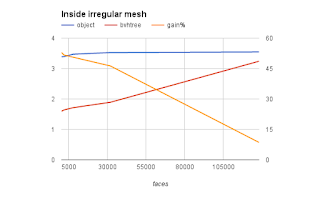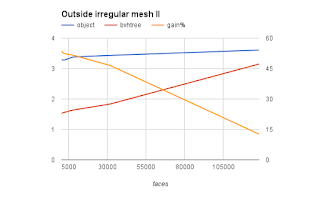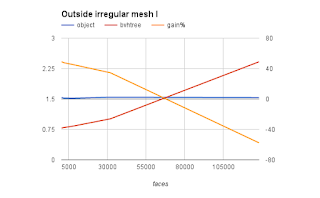### Performance of ray casting in Blender Python

The Python API for ray casts on objects and scenes was changed in a breaking manner in Blender 2.77. And because several of my add-ons depend on it, I not only had to fix them but I decided to have a look at the performance as well.
The central use case for ray casting in these add-ons is to check whether a point lies inside a mesh. This is determined by casting a ray and checking if the number of intersections is even or odd (where we count 0 as even). An even number of crossings means the point lies outside the mesh (true for the red points in the illustration) while an odd number means that the point lies inside the mesh (the green point). And the fun part is that this is true for any direction of the ray!

## Code

We can easily convert this to Python using Blenders API: given an object and point to test, we take some direction for our ray (here right along the x-direction) and check if there is an intersection. If so, we we test again but start at the intersection point, taking care to move a tiny bit along the ray cast direction to avoid getting 'stuck' at the intersection point due to numerical imprecision. We count the number of intersections and return True if this number is odd.

```import bpy
from mathutils import Vector
from mathutils.bvhtree import BVHTree

def q_inside(ob, point_in_object_space):
direction = Vector((1,0,0))
epsilon = direction * 1e-6
count = 0
result, point_in_object_space, normal, index = ob.ray_cast(point_in_object_space, direction)
while result:
count += 1
result, point_in_object_space, normal, index = ob.ray_cast(point_in_object_space + epsilon, direction)
return (count % 2) == 1
```

### Intersection with an object

We can now use this function to determine if a point lies within a mesh object:
```        q_inside(ob, (0,0,0))
```
Note that the point to check is in object space so if you want to check if a point in world space lies within the mesh you should convert it first using the inverted wordl matrix of the object.

### Intersection with a precalculated BVH tree

To determine if a ray hits any of the possibly millions of polygons that make up a mesh, the ray_cast() method internally constructs something called a BVH tree. This is a rather costly operation but fortunately a BVH tree is cached so when we perform many intersection tests this overhead should pay off. BVH trees however can also be constructed from a mesh object separately and offers exactly the same signature ray_cast() method. This means we can perform the same check to see if a point lies inside a mesh with following code:
```    sc = bpy.context.scene

bvhtree = BVHTree.FromObject(ob, sc)

q_inside(bvhtree, (0,0,0))```

## Performance

When doing millions of tests on the same mesh a small amount of overhead averages out so the question is if it is meaningful to create a separate BVH tree. If we look at the time it takes to do a million tests for meshes of different sizes we see that the overhead apparently is not the same but for larger meshes this difference loses significance:

### inside irregular mesh (subdivided Suzanne)

facesobjectbvhtreegain%
1280003.5473.2418.6
320003.5261.89246.3
80003.4731.71550.6
20003.3931.63951.7
5003.3911.59752.9### outside irregular mesh with mostly immediate initial hit

The initial data was for a point inside a mesh: such a point will always need at least two ray casts: the first will intersect some polygon, the second will veer off into infinity. If we test a point outside a mesh we sometimes will have only one ray cast and sometimes two. if we look at the latter scenario we see similar behavior:
facesobjectbvhtreegain%
1280003.6083.14812.7
320003.4341.83646.5
80003.3811.63751.6
20003.2771.55852.4
5003.2941.52853.6### outside irregular mesh with mostly no initial hits

A strange thing happens when the point to test is outside the mesh but the ray happens to point away from the mesh so that we will have zero intersections. Now for large meshes the overhead of a separate BVH tree appears larger!
facesobjectbvhtreegain%
1280001.5342.417-57.6
320001.5441.01134.5
80001.520.83245.2
20001.5230.79647.7
5001.5340.78349## Conclusion

i cannot quite explain the behavior of the ray_cast() performance for larger meshes when the point is outside the mesh and the test ray points away, but in arbitrary situations and moderately sized meshes a perfomance gain of 40% for the BVH tree is worthwhile when doing millions of tests.